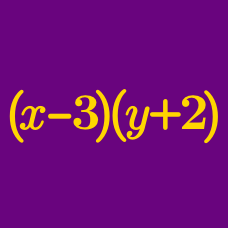Algebra

# Simplifying Expressions Warmup

What is the value of this expression?

$\frac{x}{4}\times\frac{3}{x}\times\frac{2}{y}\times\frac{y}{3}\times\frac{4}{7}$

If $a(3x^2 - 4x + 2) = -6x^2 + 8x - 4,$ what is the value of $a?$

What is the quotient?

$(-4x^3 + 8x^2 - 20x) \div (-4x)$

The perimeter of a rectangle is $18x + 2.$ If the rectangle's length is $6x + 2,$ what is the width?

Which expression is not equivalent to $(3x^3y^3)^3?$

×